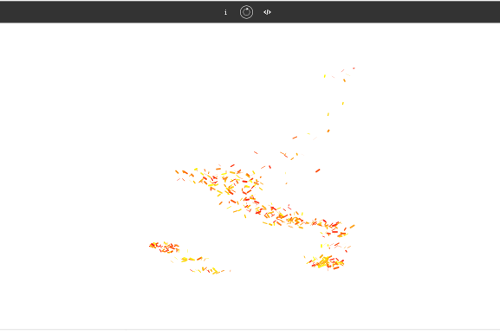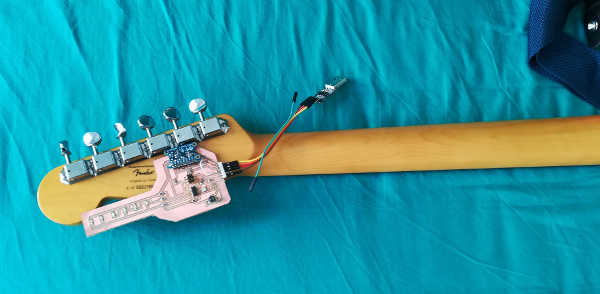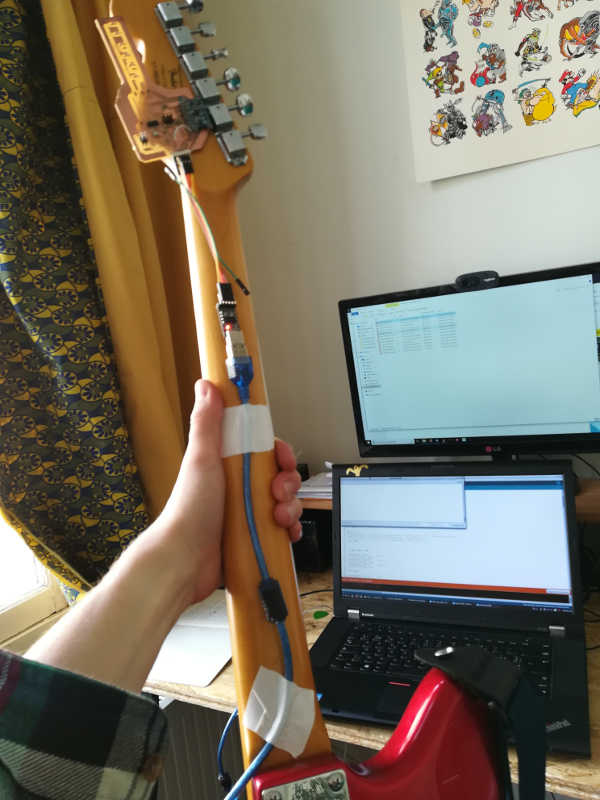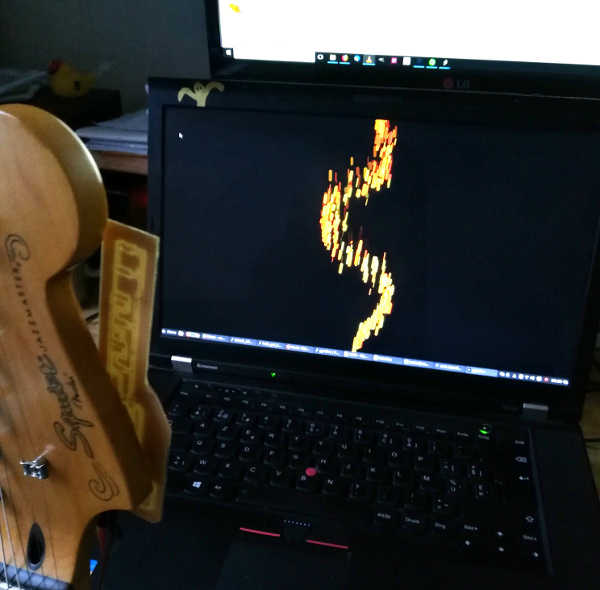Interface and Application Programming

The assigment page
write an application that interfaces with an input &/or output device that I made.

Files:
-Arduino sketch
-Processing sketch

This week I managed to make the gyro work. You can read all the explanations on my updated Input device page.
For this week's assigment, I used Arduino and Processing in order to transform my guitar into a flamethrower.

Arduino sketch
The goal was to read gyroscope's value using serial communication. I used a script I wrote for Output devices. It's mixing Input and Output:I change those lines in order to have only numbers and Z values (lines between 37 and 41):

gyro.read(); // serial.print("X: "); serial.print((int)gyro.data.x); serial.print(" "); //Data's serial prints // serial.print("Y: "); serial.print((int)gyro.data.y); serial.print(" "); /* serial.print("Z: ");*/ serial.println((int)gyro.data.z); serial.print(" "); delay(100); I uploaded the code into the board and managed to read only Z values.

Processing part
For processing, I searched a bit to find a sketch for generating fire. I find this one on OpenProcessing:Flames appears when you're pressing any of your mouse's button. The original is like this: ArrayList embers; void setup() { size(500, 500); embers = new ArrayList(); frameRate(30); } void draw() { println(embers.size()); if(mousePressed) for (int i=0; i<20; i++) embers.add(new Ember()); //Adds an ember near the cursor position. background(255); for (int i=embers.size()-1; i>=0; i--) { Ember p = (Ember) embers.get(i); p.display(); //displays the embers if (p.y<0||random(0, 30)>29) //deletes off-screen embers, and deletes "cold" embers embers.remove(i); } } class Ember { float x; float y; Ember() { x = mouseX+random(-30, 30); y = mouseY+random(-3, 3); } void display() { strokeWeight(random(0, 4)); stroke(random(250, 255), random(30, 255), random(0, 35)); float xMove = random(-10, 10); float yMove = sqrt(random(0, 31)); line(x, y, x+xMove, y-yMove); x+=xMove; y-=yMove; } } The final one is like this:
It's simpler than the original one because I removed the MousePressed condition in order to have a constant flame's flow.
And I simply link the X position of the flame to the Z serial values from the gyro. ArrayList embers; //Setting serial communication import processing.serial.*; Serial myPort; // Create object from Serial class String val = "in"; int val_aux = 0; float val_map =0; void setup() { // size(1600, 900); //I decided to make the sketch fullScreen fullScreen(); myPort = new Serial(this, "/dev/ttyUSB0", 9600); embers = new ArrayList(); frameRate(30); } void draw() { if ( myPort.available() > 0) { // If data is available val = myPort.readStringUntil('\n'); val = trim(val); if (val == null){ val = "OPS"; } else { val_aux = Integer.parseInt(val); } } println(val_aux); // println(embers.size()); //I remove "if(mousePressed)". Flames are always diplayed. for (int i=0; i<20; i++) embers.add(new Ember()); //Adds an ember near the cursor position. background(0); for (int i=embers.size()-1; i>=0; i--) { Ember p = (Ember) embers.get(i); p.display(); //displays the embers if (p.y<0||random(0, 30)>29) //deletes off-screen embers, and deletes "cold" embers embers.remove(i); } } class Ember { float x; float y; Ember() { x = 1600/2; y = 900; } void display() { //Flames are bigger strokeWeight(random(0, 14)); stroke(random(250, 255), random(30, 255), random(0, 35)); //xMove is coordonate with Z values from gyro here: float xMove = random(val_aux*2, val_aux*3); float yMove = sqrt(random(0, 1000)); line(x, y, x+xMove, y-yMove); x+=xMove; y-=yMove; } } I fixed my board to my guitar's neck and connect it to the computer with an USB cable extension:And this is the result:And here's the result in situation using my projector: